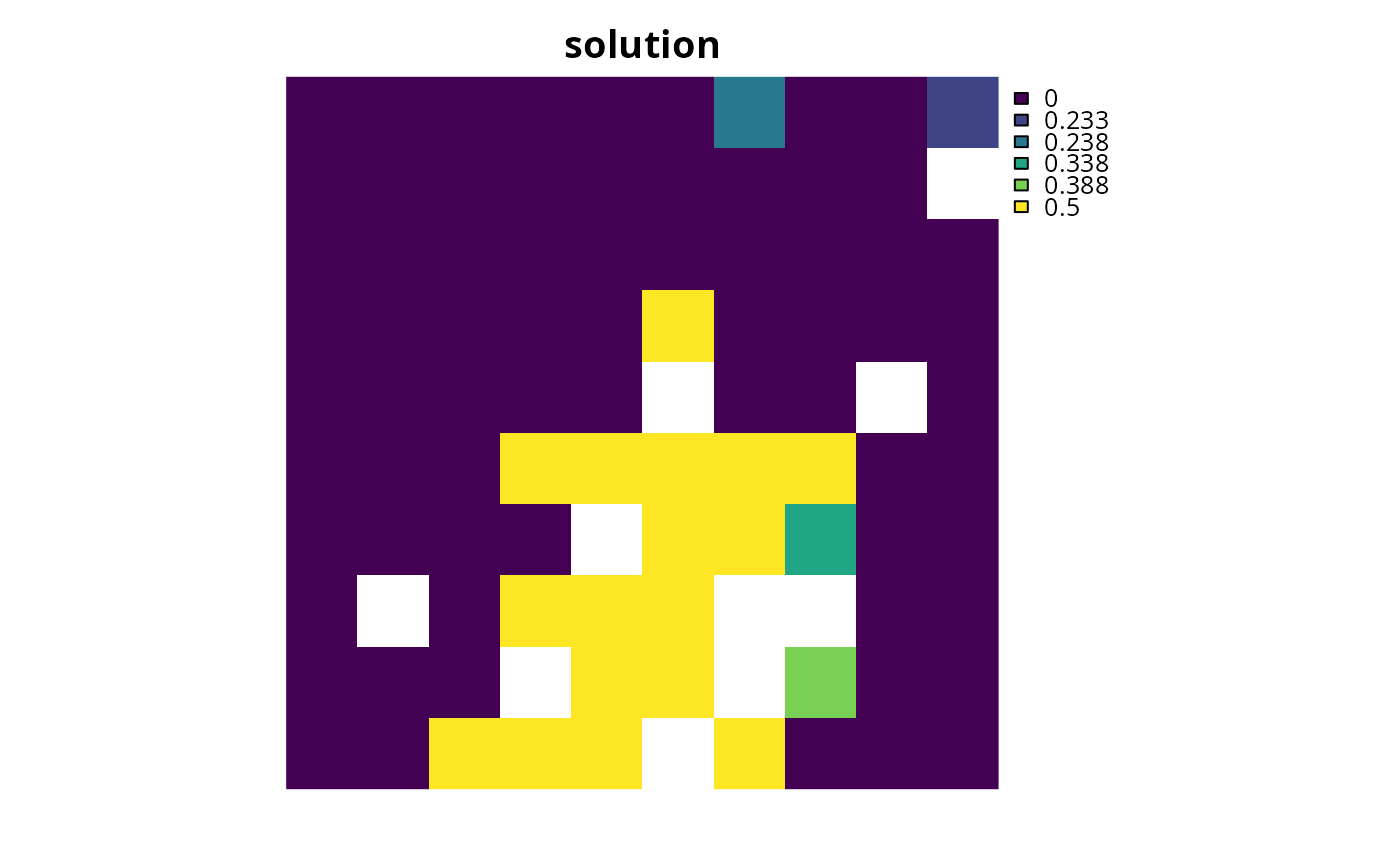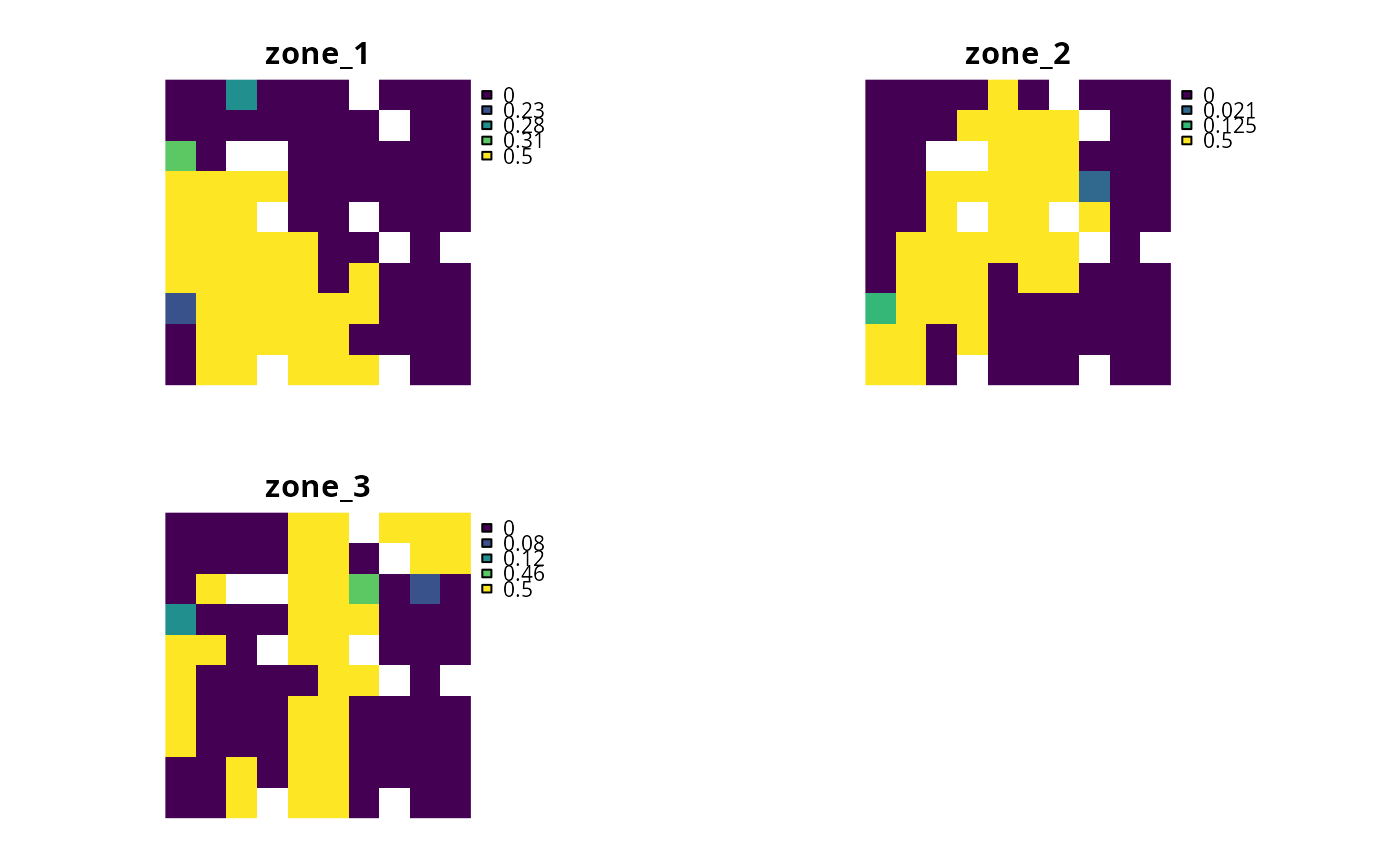Add a semi-continuous decision to a conservation planning problem. This is a relaxed decision where a part of a planning unit can be prioritized, as opposed to the entire planning unit. This decision is similar to the add_proportion_decisions() function, except that it has an upper bound parameter. By default, the decision can range from prioritizing none (0%) to all (100%) of a planning unit. However, an upper bound can be specified to ensure that, at most, only a fraction (e.g., 80%) of a planning unit can be prioritized. This type of decision may be useful when it is not practical to conserve entire planning units.

## Usage

add_semicontinuous_decisions(x, upper_limit)

## Arguments

x

problem() object.

upper_limit

numeric value specifying the maximum proportion of a planning unit that can be reserved (e.g., set to 0.8 for 80%).

## Value

An updated problem() object with the decisions added to it.

## Details

Conservation planning problems involve making decisions on planning units. These decisions are then associated with actions (e.g., turning a planning unit into a protected area). Only a single decision should be added to a problem() object. Note that if multiple decisions are added to an object, then the last one to be added will be used.

See decisions for an overview of all functions for adding decisions.

Other decisions: add_binary_decisions(), add_proportion_decisions()

## Examples

# \dontrun{
# set seed for reproducibility
set.seed(500)

sim_pu_raster <- get_sim_pu_raster()
sim_features <- get_sim_features()
sim_zones_pu_raster <- get_sim_zones_pu_raster()
sim_zones_features <- get_sim_zones_features()

# create minimal problem with semi-continuous decisions
p1 <-
problem(sim_pu_raster, sim_features) %>%

# solve problem
s1 <- solve(p1)

# plot solutions
plot(s1, main = "solution", axes = FALSE)# build multi-zone conservation problem with semi-continuous decisions
p2 <-
problem(sim_zones_pu_raster, sim_zones_features) %>%
add_relative_targets(matrix(runif(15, 0.1, 0.2), nrow = 5, ncol = 3)) %>%

# solve the problem
s2 <- solve(p2)

# print solution
print(s2)
#> class       : SpatRaster
#> dimensions  : 10, 10, 3  (nrow, ncol, nlyr)
#> resolution  : 0.1, 0.1  (x, y)
#> extent      : 0, 1, 0, 1  (xmin, xmax, ymin, ymax)
#> coord. ref. : Undefined Cartesian SRS
#> source(s)   : memory
#> names       : zone_1, zone_2, zone_3
#> min values  :    0.0,    0.0,    0.0
#> max values  :    0.5,    0.5,    0.5

# plot solution
# panels show the proportion of each planning unit allocated to each zone
plot(s2, axes = FALSE)# }